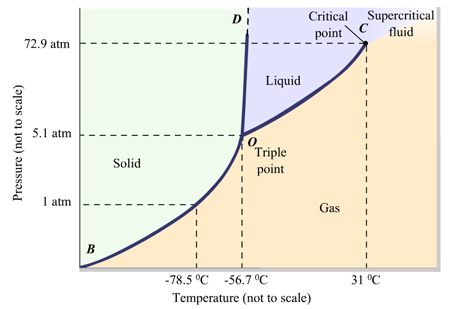Problem: A phase diagram is a pressure-temperature graph that shows the ranges of temperature and pressure over which each phase is stable. The phase diagram is divided into three regions, each region representing one stable phase: solid, liquid, or gas. There are three boundary lines that separate the phases from one another. These boundary lines represent the equilibrium between two phases. The point at which the three boundary lines intersect is called the triple point. All three phases can exist in equilibrium with each other at this point. Use the phase diagram for CO 2 and determine which of the following statements are correct. SELECT ALL THAT APPLY. All three phases of CO2 exist simultaneously at the triple point. When the pressure is 1 atm, there is no temperature at which the liquid phase of CO  2 exists. CO2 forms a supercritical fluid at temperatures less than 31 degrees Celsius. CO2 is a gas under normal conditions of temperature and pressure. Movement across boundary line BO corresponds to a phase change. When the pressure is 4 atm and the temperature is more than -56.7 degrees Celsius, CO  2 exists as a solid.

FREE Expert Solution
85% (191 ratings)
Problem Details

A phase diagram is a pressure-temperature graph that shows the ranges of temperature and pressure over which each phase is stable.

The phase diagram is divided into three regions, each region representing one stable phase: solid, liquid, or gas. There are three boundary lines that separate the phases from one another. These boundary lines represent the equilibrium between two phases. The point at which the three boundary lines intersect is called the triple point. All three phases can exist in equilibrium with each other at this point.

Use the phase diagram for CO 2 and determine which of the following statements are correct.

SELECT ALL THAT APPLY.

• All three phases of CO2 exist simultaneously at the triple point.
• When the pressure is 1 atm, there is no temperature at which the liquid phase of CO  2 exists.
• CO2 forms a supercritical fluid at temperatures less than 31 degrees Celsius.
• CO2 is a gas under normal conditions of temperature and pressure.
• Movement across boundary line BO corresponds to a phase change.
• When the pressure is 4 atm and the temperature is more than -56.7 degrees Celsius, CO  2 exists as a solid.What scientific concept do you need to know in order to solve this problem?

Our tutors have indicated that to solve this problem you will need to apply the Phase Diagrams concept. You can view video lessons to learn Phase Diagrams Or if you need more Phase Diagrams practice, you can also practice Phase Diagrams practice problems .

What is the difficulty of this problem?

Our tutors rated the difficulty of A phase diagram is a pressure-temperature graph that shows t... as medium difficulty.

How long does this problem take to solve?

Our expert Chemistry tutor, Dasha took 6 minutes to solve this problem. You can follow their steps in the video explanation above.

What professor is this problem relevant for?

Based on our data, we think this problem is relevant for Professor Donadio's class at UCD.